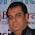# GATE-Computer Networks - Token Ring

Previous GATE questions with solutions on Computer Networks (Token Ring) - CS/IT

GATE - 2007
1. In a token ring network the transmission speed is 10bps and the propagation speed is 200 metres/ms. The 1-bit delay in this network is equivalent to:
(a) 500 metres of cable      (b) 200 metres of cable
(c) 20 metres of cable       (d) 50 metres of cable

Ans: option (c)
Explanation:
Transmission delay for 1 bit t = 1/(107) = 0.1 micro seconds.
Given that propagation speed = 200 meters/ms
That means, 200 meters can be traveled in 1 micro second.
Hence in 0.1 micro seconds, 20 meters can be traveled.

OR

1 Bit Delay = 1/B = 1/(107) = 0.1 micro seconds.
Given that propagation speed = 200 meters/ms
That means, 200 meters can be traveled in 1 micro second.
Hence in 0.1 micro seconds, 20 meters can be traveled.

1.2.